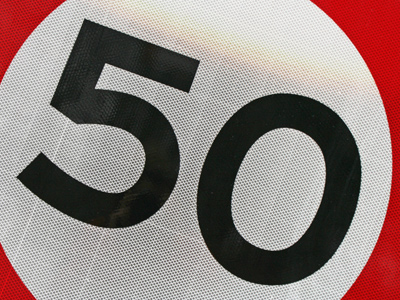10 x 5 = 50.

# 5 Times Table

Learning the times tables is a vital tool when multiplying numbers. By the end of Year 2 children are often fluent in their times tables up to 10. When they enter into KS2 in Year 3 they will also be taught some higher times tables in their Maths and Numeracy lessons. By the end of KS2 they will be familiar with all of the times tables from one to twelve. This quiz will test their knowledge of the 5 times table.

Learning the 5 times table is all about multiplying numbers by 5. This is one of the easier times tables to learn. One trick that might help you in learning the 5 times table is to multiply the numbers by 10 and then halve them. For example 4 x 5. If you multiply 4 x 10, you get 40. Half of 40 is 20. Therefore 4 x 5 must equal 20.

See how well you do in the following 5 times table quiz.

1.
8 x 5 =
35
38
40
48
2.
9 x 5 =
14
25
40
45
To multiply a number by 9 first times it by 10 and then take it away. So 5 x 10 = 50 and 50 - 5 + 45
3.
2 x 5 =
2
5
10
15
4.
6 x 5 =
11
30
35
60
5.
5 x 5 =
10
15
20
25
When a number is multiplied by itself we say that it has been squared, so 5 squared is 25
6.
12 x 5 =
55
59
60
65
To times any number by 5 just times it by 10 and then half it, so 12 x 10 = 120 and 120 ÷ 2 = 60
7.
7 x 5 =
35
40
42
45
7 x 5 is the same as (5 x 5) + (2 x 5). 5 x 5 = 25 and 2 x 5 = 10. 25 + 10 = 35 so 7 x 5 = 35
8.
4 x 5 =
9
12
16
20
4 x 5 can be either 4 + 4 + 4 + 4 + 4 or 5 + 5 + 5 + 5
9.
11 x 5 =
50
55
52
58
10.
3 x 5 =
10
12
15
18

Author:  Amanda Swift